Trees on Graphs

April 4, 2017, 11:02 pm

A couple of weeks ago, I was solving a Codeforces problem and I came across a trick I hadn't seen before. Here, I'll give that problem, describe the solution, and then show how to apply it to a more complicated problem.

Codeforces 786B: Legacy

In this problem, we are asked to compute the single source shortest paths in a weighted directed graph, with all the weights nonnegative. The catch: there are three types of edges. There are normal edges of the form $$(u, v)$$, but there are also edges that look like $$(u, [l, r])$$ and $$([l, r], u)$$. Here, $$[l, r]$$ refers to all the nodes whose index is between $$l$$ and $$r$$, inclusive. There are up to $$10^5$$ nodes and edges.

The naive solution is to convert all edges of the form $$(u, [l, r])$$ to $$r - l + 1$$ edges of the form $$(u, i)$$ (and to do the same for all edges of the form $$([l, r], u)$$). Of course, there are $$O(N \cdot M)$$ edges in this transformed graph (where $$N$$ deontes the number of nodes in the original graph, and $$M$$ is the number of edges), so this solution will MLE or TLE.

Let's digress for a minute and consider a different problem: given an array of numbers and $$Q$$ queries, each of the form $$[l, r]$$, output the minimum value in the subarray defined by each query. There are multiple solutions to this problem, but perhaps the most common is to use a segment tree: we build a binary tree on top of the array and decompose queries into $$O(\log N)$$ disjoint intervals (represented by nodes in the tree) whose union is the desired query.

For example, say the array is $$[5, 3, 5, 10, 7, 2, 8, 14]$$, and our query is $$[3, 6]$$ (we are using $$1$$-indexing). This query can be represented as the union of $$[3, 4]$$ and $$[5, 6]$$, two nodes present in our segment tree.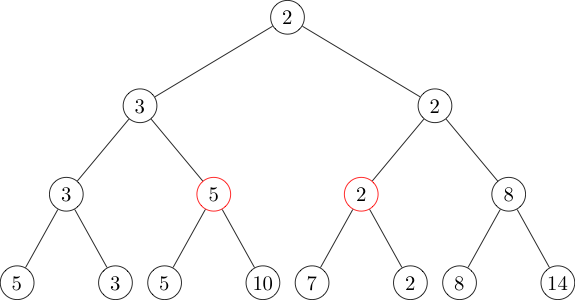The two red nodes represent the intervals $$[3, 4]$$ and $$[5, 6]$$, so if we identify them in $$O(\log N)$$ time we can then find the answer to our original query in $$O(\log N)$$ time by taking the minimum of the values in those nodes. (Exercise: prove that all intervals are the disjoint union of $$O(\log N)$$ nodes, and code a segment tree.)

Now, back to our shortest paths problem. We will use the same idea: decompose an interval $$[l, r]$$ into $$O(\log N)$$ disjoint smaller intervals on which we can do some preprocessing.

In this case, we will need two trees: one with edges directed down the tree and one with edges directed up the tree. All these edges will have weight zero.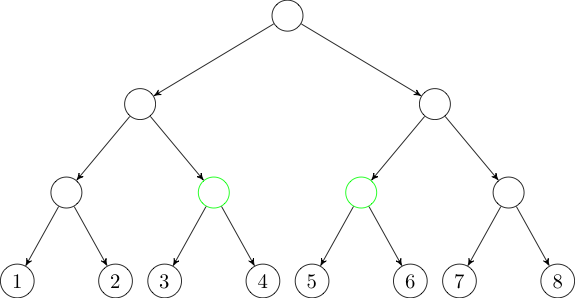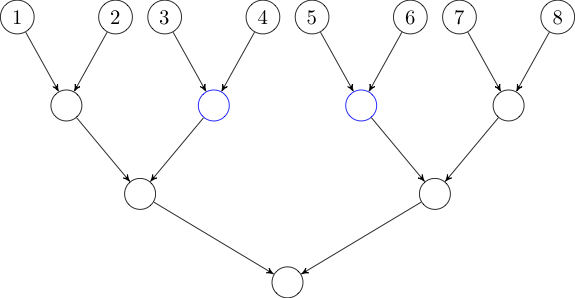Now, if we want to add an edge from some node to the interval $$[3, 6]$$, we can instead add an edge to each of the two green nodes in the first tree. Similarly, if we want to add an edge from the interval $$[3, 6]$$, we can instead add an edge from each of the two blue nodes in the second tree.

We've added $$O(M \log N)$$ edges to our graph, and running Dijkstra's algorithm on the new graph easily runs in time.

AtCoder RC069F: Flags

This problem is from a recent AtCoder contest. I didn't get to do this contest live, but I found the problems really enjoyable. Flags was the hardest problem, largely because it combines a few different techniques.

We want to place $$N$$ flags in a line while maximizing the distance between the closest two flags. Each flag has two positions in which it is allowed to be placed, and $$N$$ can be up to $$10^4$$.

We will binary search on the answer; denote our current guess $$d$$. Checking whether it is possible to place all the flags with at least distance $$d$$ between every pair is a 2-SAT problem.

A common technique for solving a 2-SAT problem like this is to build the "implication graph": a directed graph in which the nodes represent boolean variables and their negations, and edges represent logical conditionals. So an edge exists between two nodes $$u$$ and $$v$$ if $$u$$ "implies" $$v$$, and if we assign $$u$$ the truth value "true", then $$v$$ must also be marked "true". Then the 2-SAT problem is satisfiable if it is not the case that some node directly or indirectly implies its negation, and its negation directly or indirectly implies the node itself. We can easily check this condition by finding the strongly connected components of the implication graph.

So let's build the implication graph. Each flag has two nodes, one for each of its possible locations. We will assign each of these nodes a truth value, and we require that, for a solution to be valid, the two nodes associated with each must be assigned opposite truth values. That is, each flag must be placed in exactly one of its two positions. For convenience, we will say that if node $$u$$ represents placing a particular flag in its first location, then $$\neg u$$ refers to the node representing placing that flag in its second location (and vice versa).

We will add an edge from $$u$$ to $$\neg v$$ if the distance between the posistions associated with $$u$$ and $$v$$ is less than $$d$$. This edge indicates that if we assign $$u$$ "true", then all the potential positions that are nearby are disqualified: for all of those flags, we must take the other option. Implementing this naively will yield a time complexity of $$O(N^2 \log X)$$, where $$X$$ is the size of the space over which we're binary searching (probably $$[0, 10^9]$$). This is too slow.

However, if we sort all our nodes by position (breaking ties arbitrarily), then we can binary search before and after each node $$u$$ to get two intervals of nodes. We want to add an edge from $$u$$ to the negation of every node in those intervals.

That we're adding edges to intervals is a big hint to use the tree-on-graph technique we used in the previous problem. If we can use that trick here, we will reduce our number of edges from $$O(N^2)$$ to $$O(N \log N)$$. And in fact, the trick works the same way here, with an extra hiccup. Our tree's leaves shouldn't connect directly to the nodes they represent, but rather to their negations. The implementation is still straightforward, but this shows that we can use the tree-on-graph trick even when the ordering of nodes we use to build the tree is different than the ordering we use to decide what intervals to query when we add additional edges.

Consider the following sample case.

4
1 3
2 5
1 9
3 8


There are four flags. The first one can be placed at position $$1$$ or $$3$$, the second can be placed at $$2$$ or $$5$$, the third can be placed at $$1$$ or $$9$$, and the fourth can be placed at $$3$$ or $$8$$.

First, we will create our 2-SAT nodes. $$s$$ will be the node representing placing the first flag at position $$1$$, and $$\neg s$$ will be placing the first flag at position $$3$$. The same pattern will be used for $$t$$, $$\neg t$$, $$u$$, $$\neg u$$, $$v$$, and $$\neg v$$.

Below are the nodes in their natural order.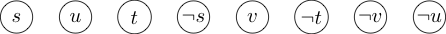Next, we will build our tree so that we can add edges to intervals. Note that the leaves of the tree are the negations of the nodes in the natural order above.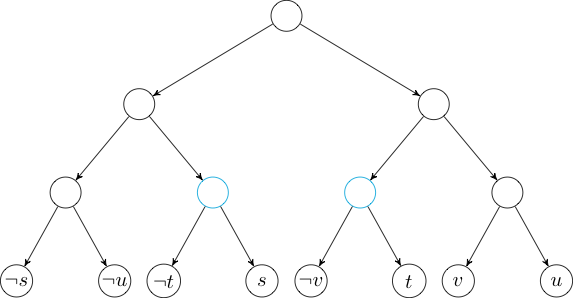Now, if we want to add an edge to the negations of the node in the interval $$[3, 6]$$, we can just add an edge to each of the nodes marked in cyan.

This completes the solution to the problem. There are $$O(N \log N)$$ edges in our new graph, and we can build the implication graph, find its strongly connected components, and ensure that no node is in the same SCC as its negation.

In this problem, the "trees on graphs" technique was only a small part of the whole solution, but for me it was the hardest part to see. In general, this technique can be used whenever we have a graph where some edges are to or from intervals of the nodes (under some ordering). The only caveat is that we must ensure that we preserve the properties of the graph we need for our solution. In the first problem, we needed to preserve path costs so that Dijkstra's algorithm would work. In the second problem, we needed to preserve the implication relationships between nodes: if (and only if) node $$u$$ is reachable from node $$v$$ in the original graph, $$u$$ should be reachable from $$v$$ in the transformed graph.

Keeping that in mind, I'm sure this can be applied to many other problems. I look forward to solving more of them in the future!